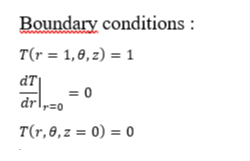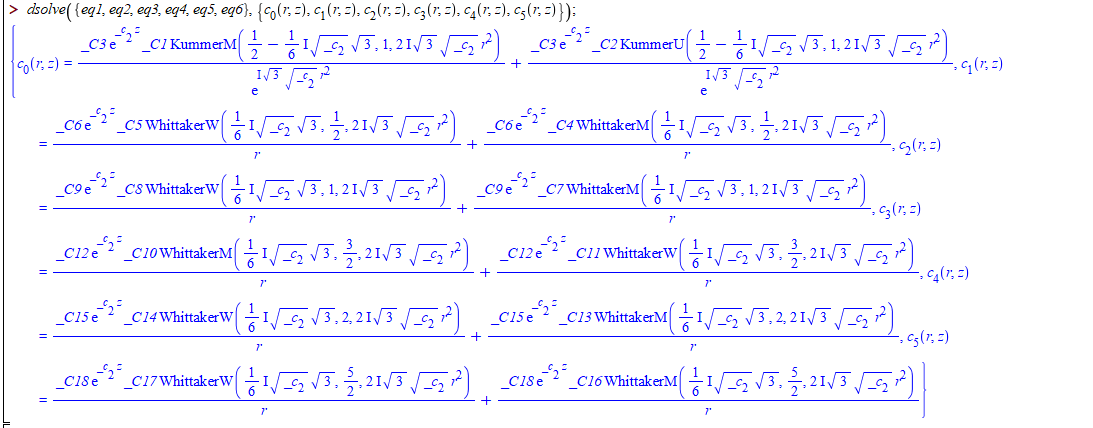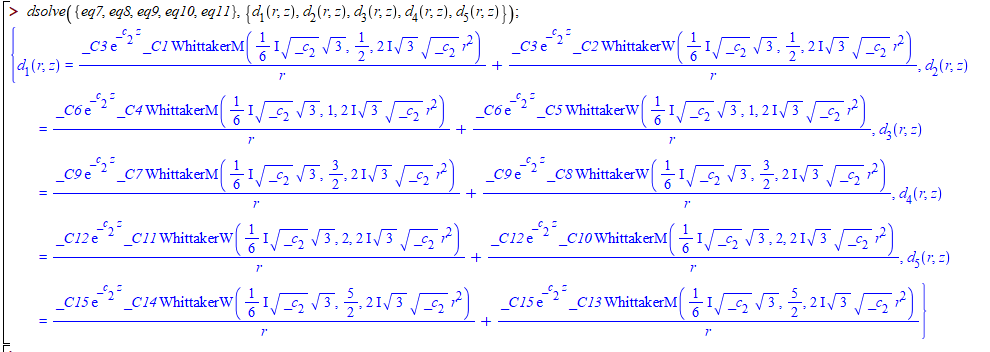## Numerical evaluation of the Zeta function...

Hello everybody – It looks like there is a bug in the numerical evaluation of (multiple) Zeta function. Take for instance, Zeta(3, 0.5) which is approximately 8.4144. Maple gives approximaterly -96.0033. Is there a bug somewhere?

Thank you

## Maximum value of a function...

Hi Users!

Hope everyone is fine here. I want to find the values of M and L for any functions f(x,y) and y(x) such that

abs(diff(f, y))       for all a ≤ x ≤ b, -∞ < y < ∞ and

abs(diff(y, x, x, x))   for all a ≤ x ≤ b, -∞ < y < ∞

For example for

f:=y-x^(2)+1; 0 ≤ x ≤ 2, -∞ < y < ∞ and

y:=(x+1)^2-.5*exp(x); 0 ≤ x ≤ 2, -∞ < y < ∞

the values of L = 1 and M = 0.5exp(2)

## How to solve a symbolic system of 25 independently...

While solving a math problem, one has to deal with a system of 25 linear equations with a parameter s (Laplace transform variable). i tried formulating the system in a matrix form using GenerateMatrix and inverting the system using solve with LU or QR method but without success. i attach a minimal working environment for the system of equations at hand. Any help or advice is highly appreciated – thank you!

## Construction of a square matrix using 2-dimesional...

Dear Users!
First, I define the following polynomial as:

restart; with(LinearAlgebra); nu := 1/2; M1 := 3; M2 := 3; #(any value of M1 and M2)
for k1 from 0 while k1 <= M1-1 do for k2 from 0 while k2 <= M2-1 do
SGP[M2*k1+k2+1] := simplify(sum((-1)^(k1-i1)*GAMMA(k1+i1+2*nu)*GAMMA(nu+1/2)*x^i1*(sum((-1)^(k2-i2)*GAMMA(k2+i2+2*nu)*GAMMA(nu+1/2)*t^i2/(GAMMA(i2+nu+1/2)*factorial(k2-i2)*factorial(i2)*GAMMA(2*nu)), i2 = 0 .. k2))/(GAMMA(i1+nu+1/2)*factorial(k1-i1)*factorial(i1)*GAMMA(2*nu)), i1 = 0 .. k1))
end do end do;
Lambda := `<,>`(seq(SGP[i], i = 1 .. M1*M2));

then, I want to define a square matrix A of order M1M2 by M1M2 after collocating SGP[k](x,y) at x=(i-1)/(M1-1) and y=(j-1)/(M2-1) for i=1,2,3,...M1, j=1,2,3,...M2.

For example, for M1=2 and M3=3 this matrix A is given as:

A:=Matrix(6, 6, {(1, 1) = SGP(0, 0), (1, 2) = SGP(0, 0), (1, 3) = SGP(0, 0), (1, 4) = SGP(0, 0), (1, 5) = SGP(0, 0), (1, 6) = SGP(0, 0), (2, 1) = SGP(0, 1), (2, 2) = SGP(0, 1), (2, 3) = SGP(0, 1), (2, 4) = SGP(0, 1), (2, 5) = SGP(0, 1), (2, 6) = SGP(0, 1), (3, 1) = SGP(1/2, 0), (3, 2) = SGP(1/2, 0), (3, 3) = SGP(1/2, 0), (3, 4) = SGP(1/2, 0), (3, 5) = SGP(1/2, 0), (3, 6) = SGP(1/2, 0), (4, 1) = SGP(1/2, 1), (4, 2) = SGP(1/2, 1), (4, 3) = SGP(1/2, 1), (4, 4) = SGP(1/2, 1), (4, 5) = SGP(1/2, 1), (4, 6) = SGP(1/2, 1), (5, 1) = SGP(1, 0), (5, 2) = SGP(1, 0), (5, 3) = SGP(1, 0), (5, 4) = SGP(1, 0), (5, 5) = SGP(1, 0), (5, 6) = SGP(1, 0), (6, 1) = SGP(1, 1), (6, 2) = SGP(1, 1), (6, 3) = SGP(1, 1), (6, 4) = SGP(1, 1), (6, 5) = SGP(1, 1), (6, 6) = SGP(1, 1)});

for M1=3 and M=2 the matrix A is given as:

A:=Matrix(6, 6, {(1, 1) = SGP(0, 0), (1, 2) = SGP(0, 0), (1, 3) = SGP(0, 0), (1, 4) = SGP(0, 0), (1, 5) = SGP(0, 0), (1, 6) = SGP(0, 0), (2, 1) = SGP(0, 1/2), (2, 2) = SGP(0, 1/2), (2, 3) = SGP(0, 1/2), (2, 4) = SGP(0, 1/2), (2, 5) = SGP(0, 1/2), (2, 6) = SGP(0, 1/2), (3, 1) = SGP(0, 1), (3, 2) = SGP(0, 1), (3, 3) = SGP(0, 1), (3, 4) = SGP(0, 1), (3, 5) = SGP(0, 1), (3, 6) = SGP(0, 1), (4, 1) = SGP(1, 0), (4, 2) = SGP(1, 0), (4, 3) = SGP(1, 0), (4, 4) = SGP(1, 0), (4, 5) = SGP(1, 0), (4, 6) = SGP(1, 0), (5, 1) = SGP(1, 1/2), (5, 2) = SGP(1, 1/2), (5, 3) = SGP(1, 1/2), (5, 4) = SGP(1, 1/2), (5, 5) = SGP(1, 1/2), (5, 6) = SGP(1, 1/2), (6, 1) = SGP(1, 1), (6, 2) = SGP(1, 1), (6, 3) = SGP(1, 1), (6, 4) = SGP(1, 1), (6, 5) = SGP(1, 1), (6, 6) = SGP(1, 1)});

and for M1=M2=3 the matrix A should be the following form:

A:=Matrix(9, 9, {(1, 1) = SGP(0, 0), (1, 2) = SGP(0, 0), (1, 3) = SGP(0, 0), (1, 4) = SGP(0, 0), (1, 5) = SGP(0, 0), (1, 6) = SGP(0, 0), (1, 7) = SGP(0, 0), (1, 8) = SGP(0, 0), (1, 9) = SGP(0, 0), (2, 1) = SGP(0, 1/2), (2, 2) = SGP(0, 1/2), (2, 3) = SGP(0, 1/2), (2, 4) = SGP(0, 1/2), (2, 5) = SGP(0, 1/2), (2, 6) = SGP(0, 1/2), (2, 7) = SGP(0, 1/2), (2, 8) = SGP(0, 1/2), (2, 9) = SGP(0, 1/2), (3, 1) = SGP(0, 1), (3, 2) = SGP(0, 1), (3, 3) = SGP(0, 1), (3, 4) = SGP(0, 1), (3, 5) = SGP(0, 1), (3, 6) = SGP(0, 1), (3, 7) = SGP(0, 1), (3, 8) = SGP(0, 1), (3, 9) = SGP(0, 1), (4, 1) = SGP(1/2, 0), (4, 2) = SGP(1/2, 0), (4, 3) = SGP(1/2, 0), (4, 4) = SGP(1/2, 0), (4, 5) = SGP(1/2, 0), (4, 6) = SGP(1/2, 0), (4, 7) = SGP(1/2, 0), (4, 8) = SGP(1/2, 0), (4, 9) = SGP(1/2, 0), (5, 1) = SGP(1/2, 1/2), (5, 2) = SGP(1/2, 1/2), (5, 3) = SGP(1/2, 1/2), (5, 4) = SGP(1/2, 1/2), (5, 5) = SGP(1/2, 1/2), (5, 6) = SGP(1/2, 1/2), (5, 7) = SGP(1/2, 1/2), (5, 8) = SGP(1/2, 1/2), (5, 9) = SGP(1/2, 1/2), (6, 1) = SGP(1/2, 1), (6, 2) = SGP(1/2, 1), (6, 3) = SGP(1/2, 1), (6, 4) = SGP(1/2, 1), (6, 5) = SGP(1/2, 1), (6, 6) = SGP(1/2, 1), (6, 7) = SGP(1/2, 1), (6, 8) = SGP(1/2, 1), (6, 9) = SGP(1/2, 1), (7, 1) = SGP(1, 0), (7, 2) = SGP(1, 0), (7, 3) = SGP(1, 0), (7, 4) = SGP(1, 0), (7, 5) = SGP(1, 0), (7, 6) = SGP(1, 0), (7, 7) = SGP(1, 0), (7, 8) = SGP(1, 0), (7, 9) = SGP(1, 0), (8, 1) = SGP(1, 1/2), (8, 2) = SGP(1, 1/2), (8, 3) = SGP(1, 1/2), (8, 4) = SGP(1, 1/2), (8, 5) = SGP(1, 1/2), (8, 6) = SGP(1, 1/2), (8, 7) = SGP(1, 1/2), (8, 8) = SGP(1, 1/2), (8, 9) = SGP(1, 1/2), (9, 1) = SGP(1, 1), (9, 2) = SGP(1, 1), (9, 3) = SGP(1, 1), (9, 4) = SGP(1, 1), (9, 5) = SGP(1, 1), (9, 6) = SGP(1, 1), (9, 7) = SGP(1, 1), (9, 8) = SGP(1, 1), (9, 9) = SGP(1, 1)});

Please help to define general matrix A for any values of M1 and M2. I will be very grateful for you.

## To eliminate some entries of a list that are not ...

Let L=[a1,a2,...,an] be a list of positive real numbers.

My Question:

How to write a procedure to find a minimal interval such as [b,c]

provided that this interval covers entries of L which are close together.

Example:

let L:=[8.1 , 2.03 , 3.5 , 0.05 , 4.1]. Then the output of the procedure is the interval [2.03 , 4.1].

In fact we want to eliminate some entries of L that are not close to other entries of L.

## Using toroidal in PDEchangecoords & defining inver...

Hello everybody,

i am trying to use PDEchangecoords to transform a system of differential equations from Cartesian coordinates to toroidal coordinates. However, when i use a user defined coordinate transform from toroidal to Cartesian, i don't get the initial equations. Please find attached a minimal working environment.

i would highly appreciate your hints and suggestions!

Thank you

Best regards,

F(1)I read the article "ONEOptimal: A Maple Package for Generating One-Dimensional Optimal System of Finite Dimensional Lie Algebra", and also searched out in Maplesoft website, but couldn't found. Does anyone have the package?

## I have a set of boundary conditions that i want t...

`# I solved the differential equation using a fourier series decomposting method. I found with the help of "Dsolve" the different expressions of the fourier constants and now I will apply the boundary conditions to find the expressions of its constants.`

#this my boundary conditions#this my system of equations## Histogram vector, starts from 0...

I have question how start histogram from 0? I have vector with probablity which start from 0.

I generate samples from my probablity:

pr=values of probablity

X:=RandomVariable(ProbablityTable(pr)):

A=Sample(X,1000):

Q=Histogram(A, binwidth=1,range=0..10):

...

I try to use bindwidth=-1 but it's impossible.

How start histogram from 0 for vectors in maple15?

## Cannot find integration...

Cannot find integration proplem_in_maple.mw

 >>>>>>(1)
 >(2)
 >(3)
 >>(4)
 >(5)
 >(6)
 >(7)
 >(8)
 >(9)
 >(10)
 >(11)
 >>(12)
 >(13)
 >(14)
 >(15)
 >(16)
 >## showing an arrow on a curve...

Dear Friends

I would like to know how I can show a simple arrow on a curve in a plot. For example for the simple sample y=x^2, for x>0, I want to show with an arrow in the figure that the trajectory begins from the point (0,0) and tends to come to the point (3,9). Or any other examples you want.

Thanks a lot

## solution of RootOf...

Dear Helpers

I have a RootOf such as below. My question is how can I obtain a simple answer for it, or how can I simplify it? Also, what's the difference between the case "index=1" and the cases "index=2" and "index=3"?

" RootOf(6*_Z^3+(27+3*RootOf(_Z^2*l^2+3*_Z^4-3)^2*sqrt(9-3*RootOf(_Z^2*l^2+3*_Z^4-3)^2*l^2))*_Z^2+(3*sqrt(9-3*RootOf(_Z^2*l^2+3*_Z^4-3)^2*l^2)*l^4*RootOf(_Z^2*l^2+3*_Z^4-3)^2-9*sqrt(9-3*RootOf(_Z^2*l^2+3*_Z^4-3)^2*l^2)*l^2+90*RootOf(_Z^2*l^2+3*_Z^4-3)^2*l^2-18*l^4+6*l^6*RootOf(_Z^2*l^2+3*_Z^4-3)^2-81+45*RootOf(_Z^2*l^2+3*_Z^4-3)^2*sqrt(9-3*RootOf(_Z^2*l^2+3*_Z^4-3)^2*l^2))*_Z-324-3*l^8+l^10*RootOf(_Z^2*l^2+3*_Z^4-3)^2+108*RootOf(_Z^2*l^2+3*_Z^4-3)^2*sqrt(9-3*RootOf(_Z^2*l^2+3*_Z^4-3)^2*l^2)-3*sqrt(9-3*RootOf(_Z^2*l^2+3*_Z^4-3)^2*l^2)*l^6+sqrt(9-3*RootOf(_Z^2*l^2+3*_Z^4-3)^2*l^2)*l^8*RootOf(_Z^2*l^2+3*_Z^4-3)^2-63*sqrt(9-3*RootOf(_Z^2*l^2+3*_Z^4-3)^2*l^2)*l^2+30*sqrt(9-3*RootOf(_Z^2*l^2+3*_Z^4-3)^2*l^2)*l^4*RootOf(_Z^2*l^2+3*_Z^4-3)^2+45*l^6*RootOf(_Z^2*l^2+3*_Z^4-3)^2+351*RootOf(_Z^2*l^2+3*_Z^4-3)^2*l^2-108*l^4, index = 1) "

Thank you so much!

## PDEs solve numerically...

Dear Users! I solved a PDE by using the following code.
restart;a := 1:b:= 2:l:= 1:alpha:= 1:alpha:= 3:
syspde:= [diff(u(x, t), t)-a+u(x, t)-u(x, t)^2*v(x, t)-alpha*(diff(u(x, t), x\$2)) = 0, diff(v(x, t), t)-b+u(x, t)^2*v(x, t)-alpha*(diff(v(x, t), x\$2)) = 0];
Bcs:= [u(x,0)=1,v(x,0)=1,D(u)(-l, t) = 0,D(u)(l, t) = 0,D(v)(-l, t) = 0,D(v)(l, t) = 0];
sol:=pdsolve(syspde, Bcs, numeric);
p1:=sol:-plot3d( u(x,t), t=0..1, x=-1..1, color=red);
p2:=sol:-plot3d( v(x,t), t=0..1, x=-1..1, color=blue);
Now I want to see the value of u(x, t)+diff(u(x, t), x)+diff(u(x, t), t) when x=0 and t=1. Please help me to fix the problem. Thanks in advance.

## fieldplot with a boundary...

Dear Guys

How can I plot a fieldplot with a boundary. For example I can plot

> fieldplot([y, -sin(x)-(1/10)*y], x = -1 .. 1, y = 0 .. 1);

but I want to see the field plot only in the region x^2+y^2<=1.

Thanks a lot

## Computation of all determinants of sub matrices of...

Let A be an nrxnr binary matrix. Suppose that the nxn binary matrix is obtained from the matrix A using the following code:

```n := upperbound(A)/r;
B := Matrix(n, n, 0);
for i to n do
for j to n do
B[i, j] := SubMatrix(A, [(i-1)*r+1 .. i*r], [(j-1)*r+1 .. j*r])
end do;
end do;```

In other words, the matrix B is a decomposition of matrix A by rxr binary matrices. In the rest, we want to compute all determinants of the submatrices of B in module 2 as follows:

```u := 1;
for k to n do
P := choose(n, k);
for i to nops(P) do
for j to nops(P) do
W := [];
for ii in P[i] do
for jj in P[j] do
W := [op(W), B[ii, jj]]
end do
end do;
x := `mod`(Det(convert(blockmatrix(k, k, W), Matrix)), 2);
if x = 0 then
u := 0;
i := nops(P)+1;
j := nops(P)+1;
k := n+1
end if
end do
end do;
unassign('i, j, ii, jj, W, x, P')
end do
```

In the last step, we check that if the value of all sub determinants of B in module 2, are non zero, then we announce that the block matrix B is an MDS matrix

`if u = 1 then print(MDS) else print(NoMDS) end if`

Based on the above description I wrote the following procedure:

```restart

with(LinearAlgebra);
with(linalg, blockmatrix);
with(combinat)

MDS:=proc(A::Matrix,r::integer)
local n,B,i,j,u,ii,jj,k,P,W,x;
n:=upperbound(A)/r;
B:=Matrix(n,n,0);
for i to n do
for j to n do
B[i,j]:=LinearAlgebra:-SubMatrix(A,[(i - 1)*r+1..i*r],[(j - 1)*r+1..j*r])
end do
end do;
unassign('i,j');
u:=1;
for k to n do
P:=combinat:-choose(n,k);
for i to nops(P) do
for j to nops(P) do
W:=[];
for ii in P[i] do
for jj in P[j] do
W:=[op(W),B[ii,jj]]
end do
end do;
x:=mod(Det(convert(linalg:-blockmatrix(k,k,W),Matrix)),2);
if x=0 then
u:=0;
i:=nops(P)+1;
j:=nops(P)+1;
k:=n+1
end if
end do
end do;
unassign('i,j,ii,jj,W,x,P')
end do;
if u=1 then print(MDS) else print(NoMDS) end if
end proc```

My problem is that if A is a 64x64 binary matrix and also r=8, then the procedure MDS(A,8) takes 453 seconds to run in my computer (Maple 15 on windows 7 32bit with 4G RAM).

Is it possible to optimize the procedure such that MDS(A,8) takes less than 1 minutes.

Thanks for any help

 1 2 3 4 5 6 7Last Page 1 of 47
﻿